Function Repository Resource:

# HedgesG

Computes the Hedges g statistic measuring an "effects size" difference between two lists of data

Contributed by: Seth J. Chandler
 ResourceFunction["HedgesG"][{lst1,lst2}] computes the "effects size" difference between two sets of data lst1 and lst2 by dividing the difference of the means of each set by the pooled standard deviation of both sets of data.

## Details and Options

The function will not evaluate unless lst1 and lst2 each have a length greater than one.

## Examples

### Basic Examples (2)

Compute the Hedges g statistic for two lists of data:

 In:=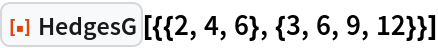Out=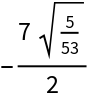Compute the Hedges g statistic of symbolic data, assuming that the elements of the data are real:

 In:=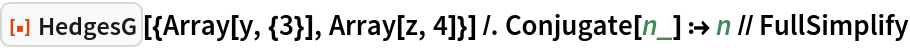Out=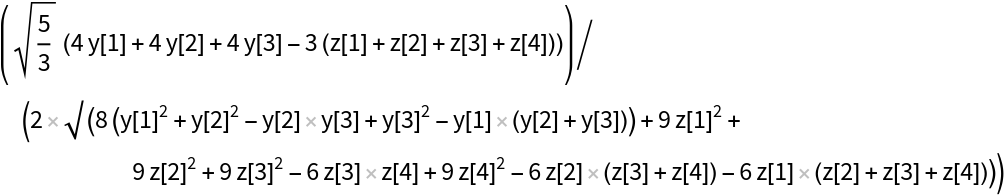### Scope (1)

The function can work on complex values, although its meaning in that case is unclear:

 In:=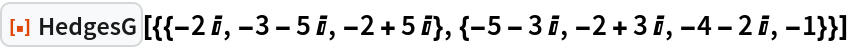Out=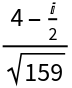### Applications (1)

Find the interval in which x must fall in order for the Hedges g statistic to exceed 0.8 (this provides a measure of when two sets of data have large differences between them):

 In:=Out=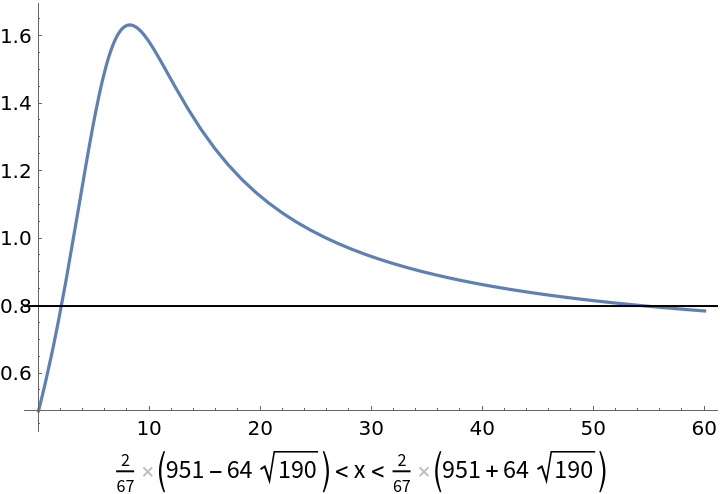### Properties and Relations (2)

The pooled standard deviation of two data samples is the difference in the means of the two samples divided by their Hedges' g statistic:

 In:=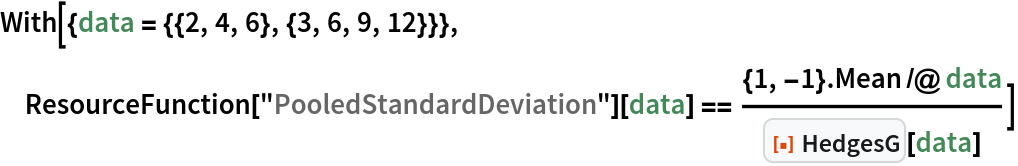Out=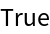The Hedges' g statistic of two lists is the negative of the Hedges g statistic of the same lists in the reverse order:

 In:=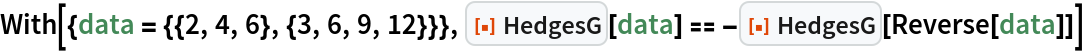Out=### Possible Issues (2)

The function will not evaluate unless each inner list has a length greater than 1:

 In:=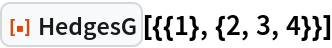Out=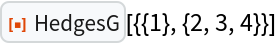The function will not evaluate if there are more than two inner lists:

 In:=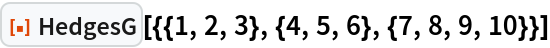Out=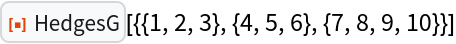Seth J. Chandler

## Version History

• 1.0.0 – 02 January 2020